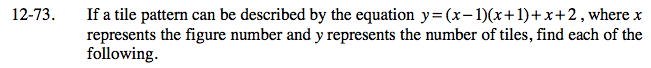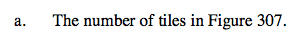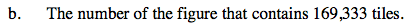### Home > CAAC > Chapter 12 > Lesson 12.4.2 > Problem12-73

12-73.Substitute 307 into the equation for x.

y = ( 307 − 1 )( 307 + 1 ) + 307 + 2

Solve for y.

y = 94,557Set y = 169,333.

169,333 = ( x − 1 )( x + 1 ) + x + 2

Solve for x.